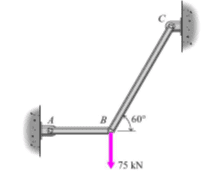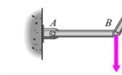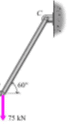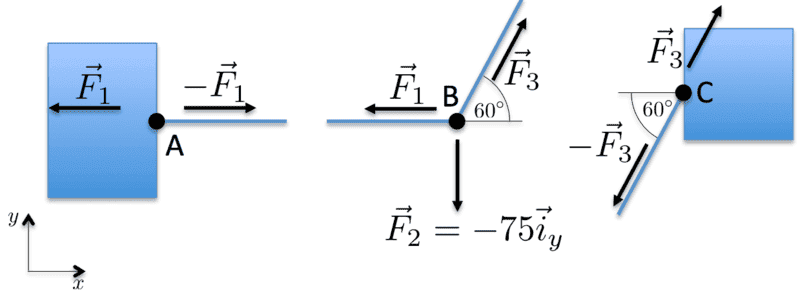# Determine the forces acting on the two rods - Free body diagram

• yellowbird321
In summary, the forces acting on the two rods in the given figure are 86.6 N and 74.3 N at points A and B respectively. The stresses present in the rods and bolts are normal stress and shear stress, which can be calculated using the equations ∑Fx = 0, ∑Fy = 0, and ∑Mx = 0. The strain can also be determined using these equations. However, further clarification is needed for the solution of rod B C and the explanation of the stresses and strain.

## Homework Statement

Determine the forces acting on the two rods in the figure. Explain which stresses that act on the rods and the bolts holding the rods to the brackets in point A and C.## Homework Equations

∑Fx = 0 ∑Fy = 0 ∑Fz = 0

∑Mx = 0 ∑My = 0 ∑Mz = 0.

## The Attempt at a Solution

∑Fx = 0: Ax – T cos 60 = 0
∑Fy = 0: Ay + sin 60 – 75 = 0
∑Mx = 0: T sin 60 - 75 = 0

75 = T sin 60
T = 75 / sin 60
T = 86,6 N

Ax = T cos 60
Ax = 86,6 cos 60
Ax = 43,3 N

Ay + sin 60 – 75 = 0
Ay = - sin 60 + 75
Ay = 74,3 N.

I have divided the figure in two: one for rod A B, and one for rod B C. I have then attempted to solve rod A B. But I am not certain how to solve for rod B C. Will it not be basically the same results? Also I am not certain where to explain the normal and shear stress, and the strain.

Starman11 said:

## Homework Statement

Determine the forces acting on the two rods in the figure. Explain which stresses that act on the rods and the bolts holding the rods to the brackets in point A and C.

View attachment 228405

## Homework Equations

∑Fx = 0 ∑Fy = 0 ∑Fz = 0

∑Mx = 0 ∑My = 0 ∑Mz = 0.

## The Attempt at a Solution

∑Fx = 0: Ax – T cos 60 = 0
∑Fy = 0: Ay + sin 60 – 75 = 0
∑Mx = 0: T sin 60 - 75 = 0

75 = T sin 60
T = 75 / sin 60
T = 86,6 N

Ax = T cos 60
Ax = 86,6 cos 60
Ax = 43,3 N

Ay + sin 60 – 75 = 0
Ay = - sin 60 + 75
Ay = 74,3 N.

I have divided the figure in two: one for rod A B, and one for rod B C. I have then attempted to solve rod A B. But I am not certain how to solve for rod B C. Will it not be basically the same results? Also I am not certain where to explain the normal and shear stress, and the strain.
Your solution is partly correct. You have done well in computing the components of the forces on point B, the tension T on rod BC and the tension Ax on rod AB. You go astray in calculating Ay. Rod AB is pivoted at points A and B and since it is horizontal, it can only exert force in the horizontal direction. So the vertical force exerted by rod AB on pivot A is zero.
You have titled your thread "Free Body diagram", but you have not drawn any free body diagrams. I suggest that you draw free body diagrams for pivot points A, B and C. That should make the solution of the problem much clearer.

Last edited:
tnich said:
Your solution is partly correct. You have done well in computing the components of the forces on point B, the tension T on rod BC and the tension Ax on rod AB. You go astray in calculating Ay. Rod AB is pivoted at points A and B and since it is horizontal, it can only exert force in the horizontal direction. So the vertical force exerted by rod AB on pivot A is zero.
You have titled your thread "Free Body diagram", but you have not drawn any free body diagrams. I suggest that you draw free body diagrams for pivot points A, B and C. That should make the solution of the problem much clearer.

Hi!

Thanks for the reply. So will this be the correct and complete solution to the problem?

Rod AB.75 = T sin 60

T = 75 / sin 60

T = 86,6 N

Ax = T cos 60

Ax = 86,6 cos 60

Ax = 43,3 N

Ay = 0

Rod BC.75 = T sin 60

T = 75 / sin 60

T = 86,6 N

Bx = T cos 60

Bx = 86,6 cos 60

Bx = 43,3 N

By + sin 60 – 75 = 0

By = - sin 60 + 75

By = 74,3 N.

#### Attachments

yellowbird321 said:
By + sin 60 – 75 = 0
It's close. The equation By + sin 60 – 75 = 0 is not correct, and your give no indication of which direction the various forces are acting. That is what you need the free body diagrams for. There are several points I would like you to take notice of with respect to the diagram below.
1) All of the information about the problem is represented in the diagram.
2) Everything is unambiguously labeled. There is no question about what any of the variables mean.
3) The rods in this problem just transmit forces, and since they only connect to pivots, those forces only act along the direction of the rods. This is a static problem, so the force on one end of a rod is countered by an equal and opposite force on the other end. The free body diagrams are useful for the pivot points.
4) If you give each force on the diagram a direction, then you can resolve the forces into x and y components with correct signs. It doesn't matter if you draw the force arrow in the wrong direction in the diagram. The algebra will still get you the right answer.
5) It's OK to draw the free body diagrams on the original picture as long as you know what the free bodies are.

Now you can write net force equations:
The net force on pivot B is
##0 = \vec F_1 + \vec F_2 + \vec F_3##

As an example, the net horizontal force on pivot B is
##0 = -F_1 + F_3 \cos(60)##

Once you solve for the horizontal and vertical components of ##\vec F_1## and ##\vec F_3##, the diagram gives you all the information you need to answer the original question about the stresses on the rods and bolts A and C.

This approach may seem like overkill for a problem like this, but practicing these techniques with simple problems will give you skills to hand complex problems. In general, drawing and labeling a diagram for each problem will save you time by organizing and recording your thoughts.#### Attachments

Hi!

Thanks for the comments. I have attemped the following:

Rod AB.
∑Fx = 0: Ax – T cos 60 = 0

∑Fy = 0: Ay + sin 60 – 75 = 0

∑Mx = 0: T sin 60 - 75 = 0

∑Mx = 0:

75 = T sin 60

T = 75 / sin 60

T = 86,6 N

∑Fx = 0:

Ax = T cos 60

Ax = 86,6 cos 60

Ax = 43,3 N

∑Fy = 0:

Ay = 0

Rod BC.
∑Fx = 0: Bx – T cos 60 = 0

∑Fy = 0: By + cos 60 – 75 = 0

∑My = 0: T sin 60 - 75 = 0

∑My = 0:

75 = T sin 60

T = 75 / sin 60

T = 86,6 N

Bx = T cos 60

Bx = 86,6 cos 60

Bx = 43,3 N

By + cos 60 – 75 = 0

By = - cos 60 + 75

By = 74,3 N.

Rod AB is pivoted at points A and B and since it is horizontal, it can only exert force in the horizontal direction. So the vertical force exerted by rod AB on pivot A is zero.

Rod BC is pivoted at points B and C and since it is vertical, it exert force in the vertical direction. So the vertical force exerted by rod BC on pivot C is 75 kN.

Is this a correct solution.

yellowbird321 said:
Rod AB is pivoted at points A and B and since it is horizontal, it can only exert force in the horizontal direction. So the vertical force exerted by rod AB on pivot A is zero.
This part is correct as far as it goes, but what is the horizontal force of rod AB on pivot A? And in which direction?

yellowbird321 said:
Rod BC is pivoted at points B and C and since it is vertical, it exert force in the vertical direction.
Rod BC is not vertical, it is at a 60 deg angle to the horizontal.

yellowbird321 said:
So the vertical force exerted by rod BC on pivot C is 75 kN.
This part is correct as far as it goes. In what direction does the force act? What is the horizontal force on pivot C, and in which direction?

What are the stresses/tensions on the rods?

I think it would help you to write out the net horizontal and vertical force equations for pivot B.

tnich said:
I think it would help you to write out the net horizontal and vertical force equations for pivot B.
I see that you have sort of done that here:

Rod AB.
∑Fx = 0: Ax – T cos 60 = 0

∑Fy = 0: Ay + sin 60 – 75 = 0

∑Mx = 0: T sin 60 - 75 = 0

but is it a bit confusing since you have not defined your variables, and this equation is still incorrect:
∑Fy = 0: Ay + sin 60 – 75 = 0

tnich said:
I see that you have sort of done that here:

but is it a bit confusing since you have not defined your variables, and this equation is still incorrect:

Rod AB.
∑Fx = 0: Ax – T cos 60 = 0

∑Fy = 0: Ay + sin 60 – 75 = 0

∑Mx = 0: T sin 60 - 75 = 0

∑Mx = 0:

75 = T sin 60

T = 75 / sin 60

T = 86,6 N

∑Fx = 0:

Ax = T cos 60

Ax = 86,6 cos 60

Ax = 43,3 N

∑Fy = 0:

Ay = 0

Rod AB is pivoted at points A and B and since it is horizontal, it can only exert force in the horizontal direction. So the vertical force exerted by rod AB on pivot A is zero.

Rod CB.

∑Fx = 0: Cx - T cos 60 = 0

∑Fy = 0: Cy - sin 60 – 75 = 0

∑My = 0: T sin 60 - 75 = 0

∑My = 0:

75 = T sin 60

T = 75 / sin 60

T = 86,6 N

Cx = T cos 60

Cx = 86,6 cos 60

Cx = 43,3 N

Cy - sin 60 – 75 = 0

Cy = sin 60 + 75

Cy = 75,9 N.